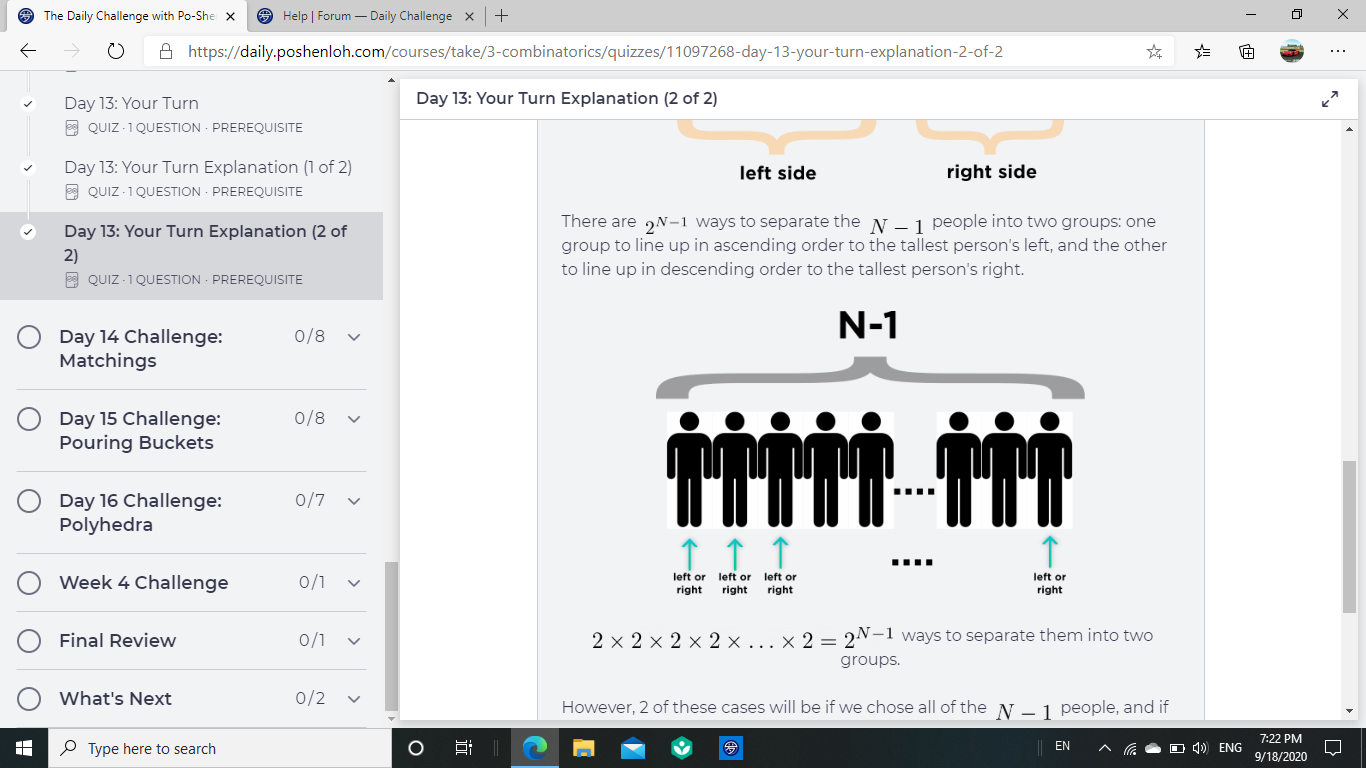# Again, how do we know for sure that it does make the other people go in ascending and descending order?

• Module 3 Week 4 Day 13 Your Turn Part 2Again, how do we know for sure that it does make the other people go in ascending and descending order?

• @The-Blade-Dancer This is a really neat trick, and you're right, it seems like we're not really caring whether the people on the sides are ascending or descending. How can this be?! It almost seems as if we're doing a different problem.

Prof. Loh's trick is to just count the number of subsets of people. Then, subtract $$2$$ for the two bad cases where the tallest person is on the end, and -- wha-la -- that's the answer!

This is because the subset that we choose can always be assigned to the left side, and placed to the left side of Mr. Tallest. The other numbers have no choice but to go on the right side. And there's only one way to line up the left people so that the tallest of them is next to Mr. Tallest, and then decreasing as we go toward the left end of the line. Similarly, there's only one way to line up the right people so that the tallest of them is next to Mr. Tallest, and they decrease as they go toward the right end of the line.

$$\textcolor{blue}{\text{ In other words, there is a one-to-one correspondence between a subset }}$$
$$\textcolor{blue}{\text{ and a complete arrangement of the people in the line which satisfies the conditions.}}$$

### Example

For example, suppose there are ten people, with names $$\{ A, B, C, D, E, F, G, H, J, \text{ and Mr. } T\},$$ Mr. $$T$$ being the tallest and $$A$$ being the shortest, with their heights increasing from $$A$$ to $$\text{ Mr. } T.$$

For any subset I choose, this corresponds to one correct arrangement of the people that you can make.Suppose you choose subset $$\{ \textcolor{red}{A}, \textcolor{red}{E}, \textcolor{red}{J} \}$$ out of the whole set $$\{ \textcolor{red}{A}, B, C, D, \textcolor{red}{E}, F, G, H, \textcolor{red}{J}, \text{ and Mr. } T\}.$$

The one correct arrangement is: $$\boxed{ \textcolor{red}{A}, \textcolor{red}{E}, \textcolor{red}{J}, \textcolor{blue}{\text{Mr. }T }, H, G, F, D, C, B. }$$Suppose you choose subset $$\{ \textcolor{red}{D, F, G, J} \}$$ out of the whole set $$\{ A, B, C, \textcolor{red}{D }, E, \textcolor{red}{F, G,} H, \textcolor{red}{J}, \text{ and Mr. } T\}.$$

The one correct arrangement is: $$\boxed{ \textcolor{red}{D, F, G, J}, \textcolor{blue}{\text{Mr. }T }, H, E, C, B, A. }$$Suppose you choose subset $$\{ \textcolor{red}{A, B, C, E, H} \}$$ out of the whole set $$\{ \textcolor{red}{A, B, C}, D, \textcolor{red}{E}, F, G, \textcolor{red}{H}, J \text{ and Mr. } T\}.$$

The one correct arrangement is: $$\boxed{ \textcolor{red}{A, B, C, E, H, } \textcolor{blue}{\text{Mr. }T }, J, G, F, D. }$$

Notice this is the flip version of the previous arrangement, so by focusing on choosing only the left-hand subset of people, we still get all of the ways, without missing any!

Basically, once you have chosen the a subset of people, there's only one way to arrange them in decreasing order, and the remaining people populate the other side. Mr. Tallest goes in between them. Subtract 2 for the ways to choose an empty subset, which puts Mr. Tallest on the end, which we don't want. Thus the number of ways to arrange $$n$$ such people is $$2^{n-1} - 2.$$